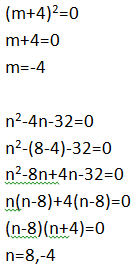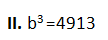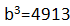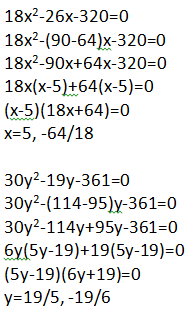New Students Offer - Use Code HELLO

# High Level Quadratic Equation Quiz Part- 7Direction (Q.1-5): In each of these questions, two equations numbered (I) and (II) are given. You have to solve both the equations and give the answer:

### Question. 1.A. m ≤ n
B. m < n
C. m ≥ n
D. m > n
E. m = n or no relationship can't be established between m and n
Ans. A.
Solution:### Question. 2.

I. (x+4)(y-5)=0
II. 4xy+60x+16y-100=0
A. x ≤ y
B. x < y
C. x ≥ y
D. x > y
E. x = y or no relationship can't be established between x and y
Ans. B.
Solution:
1. (x+4)(y-5)=0
xy-5x+4y-20=0
2. 4xy+60x+16y-100=0
Now, subtract 4 times of equation I from equation II
4xy-20x+16y-80-(4xy+60x+16y-100)=0
4xy-20x+16y-80-4xy-60x-16y+100=0
-80x+20=0Putting value of x in equation I
y=5

### Question. 3.

I. a=A. a ≤ b
B. a < b
C. a ≥ b
D. a > b
E. a = b or no relationship can't be established between a and b
Ans. E.
Solution:
a=a=17b=17

### Question. 4.A. x ≤ y
B. x < y
C. x ≥ y
D. x > y
E. x = y or no relationship can't be established between x and y
Ans. .E
Solution:### Question. 5.

I.II.A. p ≤ q
B. p < q
C. p ≥ q
D. p > q
E. p = q or no relationship can't be established between p and q
Ans. A.
Solution: